# Taylor formula

(diff) ← Older revision | Latest revision (diff) | Newer revision → (diff)

A representation of a function as a sum of its Taylor polynomial of degree() and a remainder term. If a real-valued functionof one variable istimes differentiable at a point, its Taylor formula has the form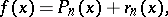where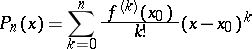is its Taylor polynomial, while the remainder term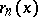can be written in Peano's form: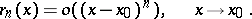If the functionistimes differentiable in some neighbourhood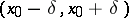,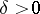, of a point, then in this neighbourhood the remainder term can be written in the Schlömilch–Roch formwhere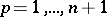; as special cases there are the Lagrange formand the Cauchy form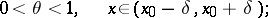the numberdepends on,and.

If the derivative of orderof the functionis integrable on the interval with end pointsand, then the remainder term can be written in integral form: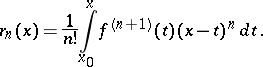Taylor's formula, with all forms of the remainder term given above, can be extended to the case of a function of several variables. Taylor's formula is also valid for mappings of subsets of a normed space into similar spaces, and in this case the remainder term can be written in Peano's form or in integral form.

Taylor's formula allows one to reduce the study of a number of properties of a function differentiable a specified number of times to the substantially simpler problems of studying these properties for the corresponding Taylor polynomial. This is the basis of various and numerous applications of the Taylor polynomial, for instance to the computation of limits of functions, to the investigation of their extreme points, their points of inflection, intervals of convexity and concavity, as well as to the convergence of series and integrals and to estimates of the speed of their convergence or divergence.